Question

# 2- D (XYZ XYZ +XYZ a. Simplify F using Boolean algebra. b. Draw the logie diagram...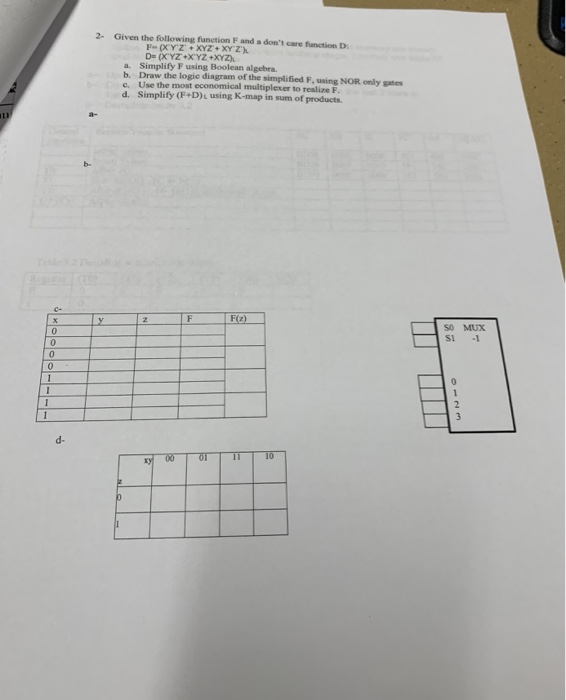2- D (XYZ XYZ +XYZ a. Simplify F using Boolean algebra. b. Draw the logie diagram of the simplified F, using NOR only gates c. Use the most economical multiplexer to realize F d. Simplify (F+D)L using K-map in sum of products so MUX si -l d-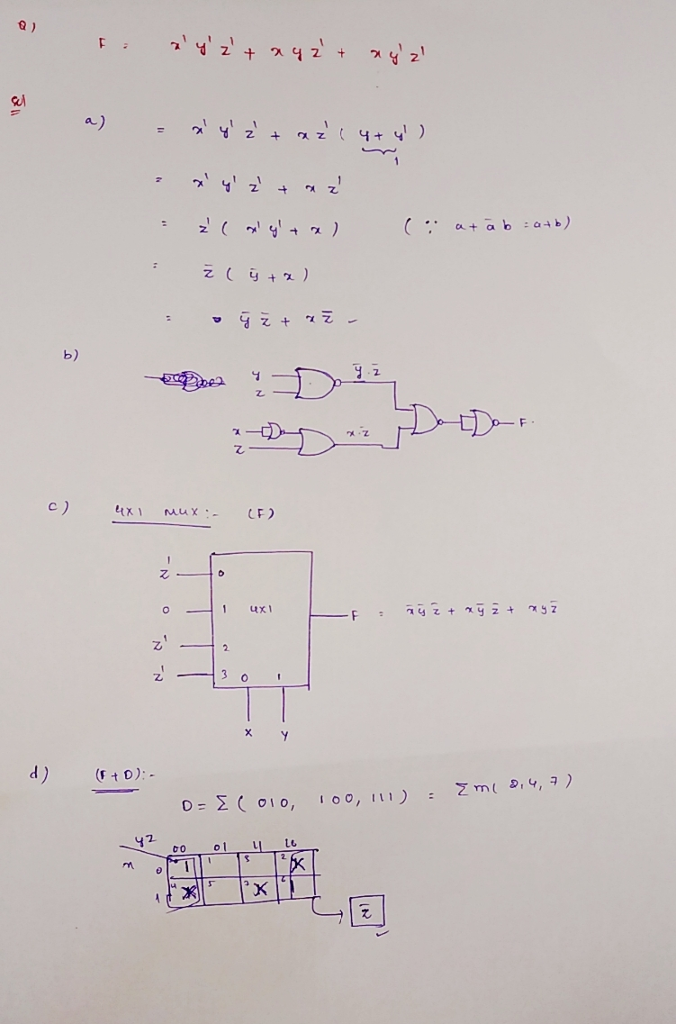#### Earn Coins

Coins can be redeemed for fabulous gifts.

Similar Homework Help Questions
• ### Q2) Given the Boolean function F = xyz + xyz + xyz (a) List the truth...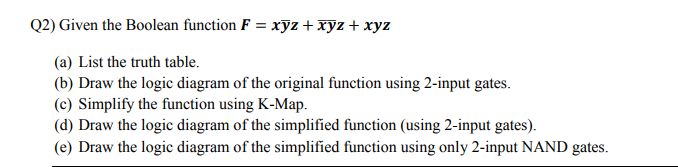Q2) Given the Boolean function F = xyz + xyz + xyz (a) List the truth table. (b) Draw the logic diagram of the original function using 2-input gates. (c) Simplify the function using K-Map. (d) Draw the logic diagram of the simplified function (using 2-input gates). (e) Draw the logic diagram of the simplified function using only 2-input NAND gates.

• ### 2.18 For the Boolean function F = xyz + x'y'z + w xy + wx'y +...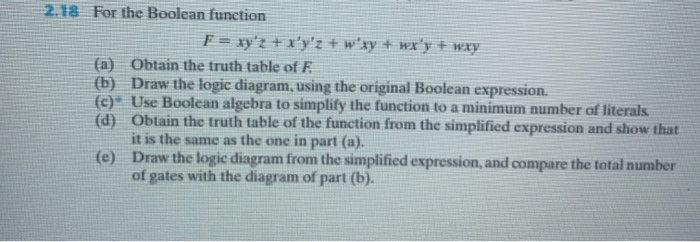2.18 For the Boolean function F = xyz + x'y'z + w xy + wx'y + wxy (a) Obtain the truth table of E (b) Draw the logic diagram, using the original Boolean expression. (c) Use Boolean algebra to simplify the function to a minimum number of literals (d) Obtain the truth table of the function from the simplified expression and show that it is the same as the one in part (a). (e) Draw the logic diagram from the...

• ### L ILLLL LLLLLLL LO LLO (7) Boolean Algebra 7 marks (7a) Simplify the following logic function...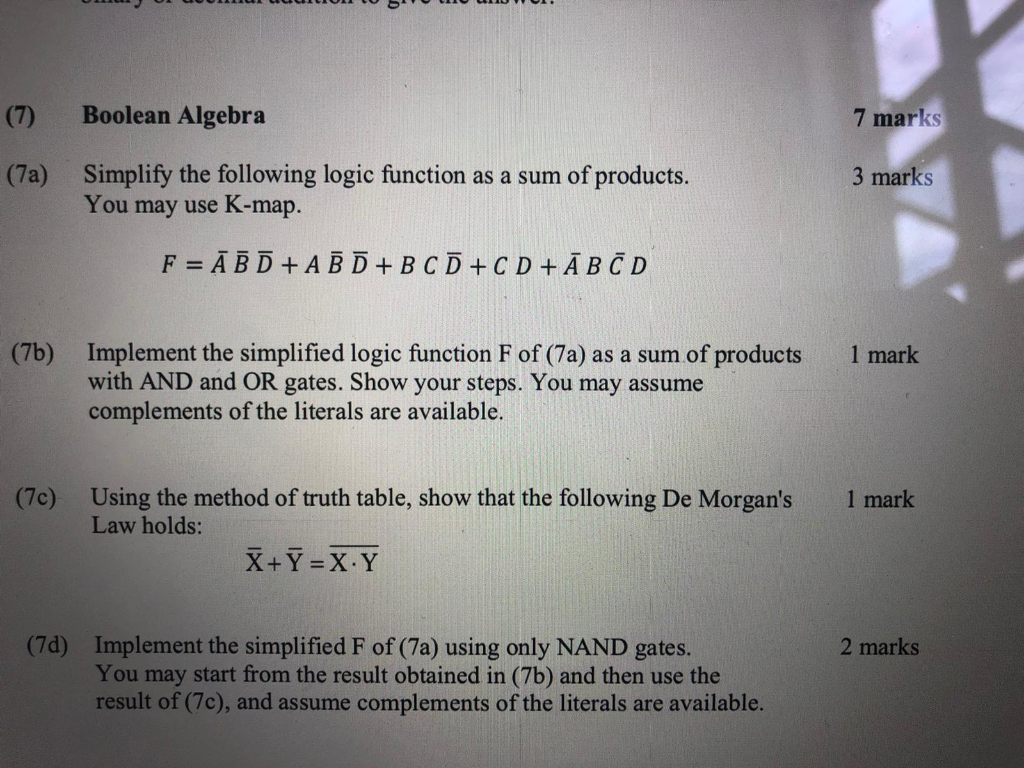L ILLLL LLLLLLL LO LLO (7) Boolean Algebra 7 marks (7a) Simplify the following logic function as a sum of products. You may use K-map. 3 marks F = Ā B D + A B D + B C D + C D + ĀB C D (76) 1 mark Implement the simplified logic function F of (7a) as a sum of products with AND and OR gates. Show your steps. You may assume complements of the literals are available....

• ### Q1: Boolean algebra 1. Simplify the following Boolean expression using Boolean algebra we learned in class...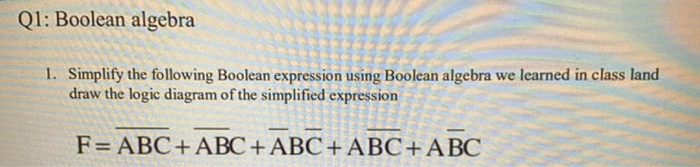Q1: Boolean algebra 1. Simplify the following Boolean expression using Boolean algebra we learned in class land draw the logic diagram of the simplified expression - - F= ABC + ABC + ABC + ABC+ ABC

• ### Given the following boolean expression: F = ABC + ABC + ABC 1. Simplify the expression...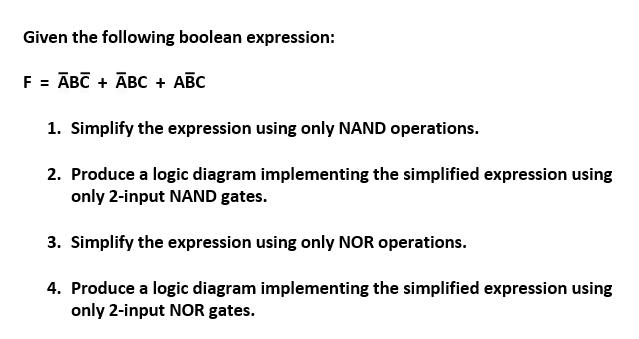Given the following boolean expression: F = ABC + ABC + ABC 1. Simplify the expression using only NAND operations. 2. Produce a logic diagram implementing the simplified expression using only 2-input NAND gates. 3. Simplify the expression using only NOR operations. 4. Produce a logic diagram implementing the simplified expression using only 2-input NOR gates.

• ### 1. (10 point 1 effort points) Simplify the Boolean function F(A, B,C, D) - 11 (3,4,6,7,1...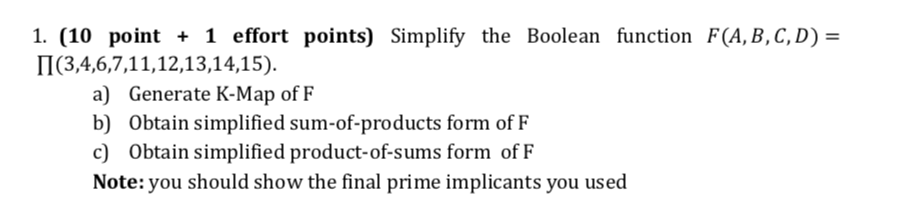1. (10 point 1 effort points) Simplify the Boolean function F(A, B,C, D) - 11 (3,4,6,7,1 1,12,13,14,15). a) Generate K-Map of F b) Obtain simplified sum-of-products form of F c) Obtain simplified product-of-sums form of F Note:you should show the final prime implicants you used

• ### 1. Simplify the following Boolean function to sum-of-product by first finding the essential prime implicant              F(A,...

1. Simplify the following Boolean function to sum-of-product by first finding the essential prime implicant              F(A, B, C, D) = ∑( 0, 1, 3, 4, 5, 7, 9, 11, 13) 2. Implement the simplified Boolean function in 1. Using NOR gates only

• ### Given the function F(x,y,z) = xyztx,y2+xyz (a) List the truth table for F (b) Draw the...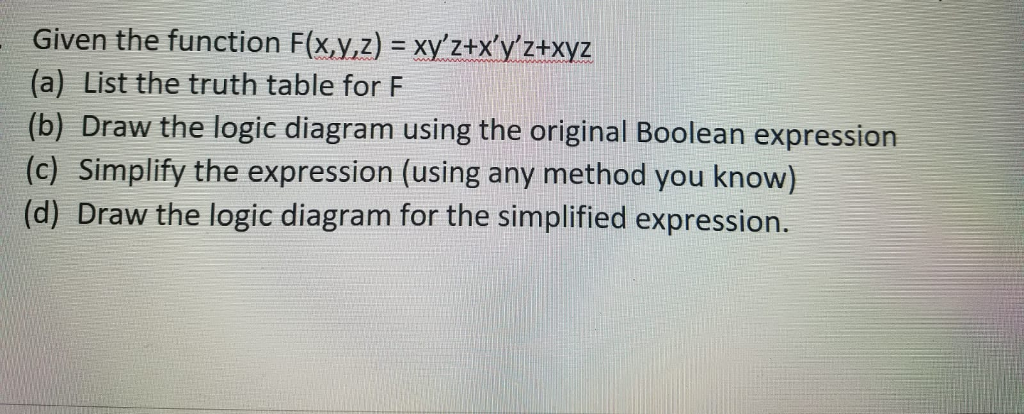Given the function F(x,y,z) = xyztx,y2+xyz (a) List the truth table for F (b) Draw the logic diagram using the original Boolean expression (c) Simplify the expression (using any method you know) (d) Draw the logic diagram for the simplified expression.

• ### CS1400 Sprin Homework 01 - Due date 1.Simplify the following functions using ONLY Boolean Algebra Theorems....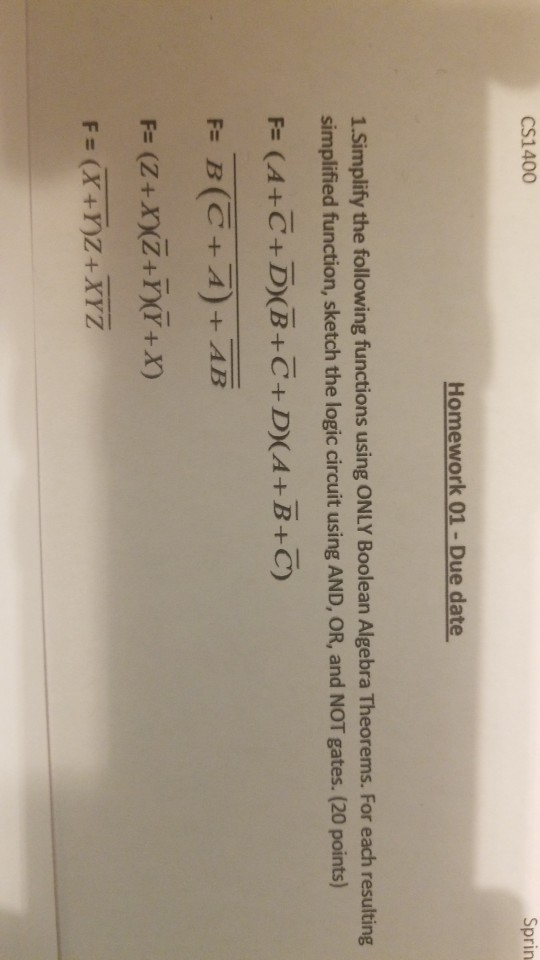CS1400 Sprin Homework 01 - Due date 1.Simplify the following functions using ONLY Boolean Algebra Theorems. For each resulting simplified function, sketch the logic circuit using AND, OR, and NOT gates. (20 points) F (A+C+ D(B+C+D(A+B+C) F B(C+A)+AB F (Z+XXZ+D+x) F= (.

• ### Q2: 1. Proof this Boolean expression. Use Boolean Algebra (X+Y). (Z+W).(X'+Y+W) = Y.Z+X.W+Y.W 2. For this...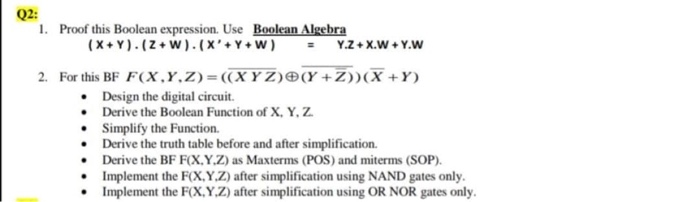Q2: 1. Proof this Boolean expression. Use Boolean Algebra (X+Y). (Z+W).(X'+Y+W) = Y.Z+X.W+Y.W 2. For this BF F(X,,Z)=((XYZ)(X +Z))(X+Y) • Design the digital circuit Derive the Boolean Function of X, Y, Z. Simplify the Function Derive the truth table before and after simplification. Derive the BF F(X,Y,Z) as Maxterms (POS) and miterms (SOP). Implement the F(X,Y,Z) after simplification using NAND gates only. Implement the F(X,Y,Z) after simplification using OR NOR gates only.